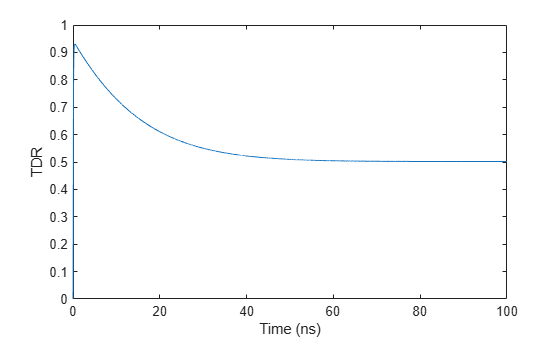Documentation

# stepresp

Step-signal response of rational function object

## Syntax

```[yout,tout] = stepresp(h, ts, n, trise) ```

## Description

`[yout,tout] = stepresp(h, ts, n, trise)` calculates the time-domain response of a rational function object, `h`, to a step signal, defined as:

`$\left\{\begin{array}{l}U\left(k{t}_{s}\right)=k{t}_{s}/{t}_{rise},\\ U\left(k{t}_{s}\right)=1,\end{array}\text{ }\text{ }\begin{array}{c}0\le k<\left({t}_{rise}/{t}_{s}\right)\\ \left({t}_{rise}/{t}_{s}\right)\le k\le N\end{array}$`

The input `h` is the handle of a rational function object returned by `rationalfit`. The variable ts is the sample time, `ts`; N is the number of samples, `n`; and trise is the time, `trise`, that it takes for the step signal to reach its maximum value. The variable k is an integer between 0 and N, referring to the index of the samples.

The following figure illustrates the construction of this signal.The output `yout` is the response of the step signal at time `tout`.

## Examples

collapse all

Calculate the step response of a rational function object from the file `passive.s2p`. Read `passive.s2p`.

```S = sparameters('passive.s2p'); freq = S.Frequencies;```

Get S11 and convert to a TDR transfer function.

```s11 = rfparam(S,1,1); Vin = 1; tdrfreqdata = Vin*(s11+1)/2;```

Fit to a rational function object.

`tdrfit = rationalfit(freq,tdrfreqdata);`

Define parameters for a step signal. Define parameters for a step signal

```Ts = 1.0e-11; N = 10000; Trise = 1.0e-10;```

Calculate the step response for TDR and plot it

```[tdr,t1] = stepresp(tdrfit,Ts,N,Trise); figure plot(t1*1e9,tdr) ylabel('TDR') xlabel('Time (ns)')```